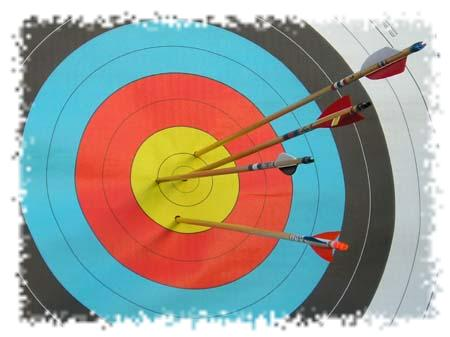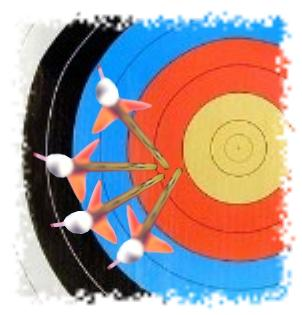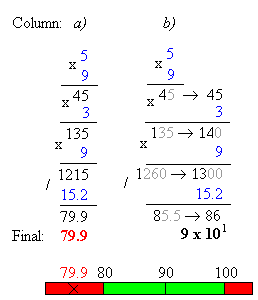# How to handle input data tolerances and how to track error propagation while performing the calculations?

"If it can't be quantified, then it's not engineering, but only a guess."

Vern Lindberg

Error in science and engineering does not equal a mistake. Rather, it represents the inevitable uncertainty that happens because of empirical measurements and cannot be perfectly corrected. All measurements in practice and even in principle have some error associated with them; no measured quantity can be determined with infinite precision and zero deviation. Without proper error analysis, no valid scientific conclusions can be drawn. In fact, wrong results can happen if error analysis is ignored.

We tend to use the words accuracy and precision interchangeably but in science and engineering they are clearly distinguished. Accuracy refers to how close a measurement is to the expected value. Using an archery target analogy where an arrow represents a measurement and the bulls-eye represents the expected or accepted value, accuracy corresponds to the distance between the arrows and the bulls-eye.Using the archery analogy, precision is the distance between each arrow, irrespective of where they lie on the target with respect to the bulls-eye. The grouping of arrows could be tightly clustered but a long way from the bulls-eye.Mathematically, accuracy is the maximum error we introduce because we truncate the digits. Therefore, accuracy refers to the number of significant digits in a number.

On the other hand, precision is the number of digits available to represent the mantissa (the part of the number after the decimal point). But beware! It is possible to have high precision with poor accuracy.

As an example, lets perform basic multiplication and division operations on real numbers (shown in blue color). We will assume that all the initial values contain significant digits only. Column a) shows step-by-step operations without consideration of margin of error of intermediate values. The column b) calculations are made according to set of rules for approximate calculations adopted by scientific community (see rules 2 and 4 below):Even this simple 4 step example proves how illusory can be the precision of the inaccurate value obtained while ignoring error propagation through computations.

ARCAD online short circuit calculator carefully handles input data tolerances and tracks error propagation through massive computations associated with short circuit analyzes. Listed are some of the rules for approximate calculations adopted by the calculator:

1. When quantities are being added or subtracted, the number of decimal places (not significant digits) in the answer should be the same as the least number of decimal places in any of the numbers being added or subtracted.
2. In calculations involving multiplication and division, the number of significant digits in an answer should equal the least number of significant digits in any one of the numbers being multiplied or divided.
3. When finding the square root of a number, the result has the same accuracy as the number
4. When doing multiple-step calculations, keep one more significant digit than required by rules above in intermediate results. This digit is dropped off the final result. In this manner, phenomenon known as "round-off error" is effectively avoided.
5. If one of the original factors has more significant digits than the other, round the more accurate number to one more significant digit than appears in the less accurate number. The extra digit protects the answer from the effects of multiple rounding.

In conventional error propagation theory, errors always increase when quantities are added, subtracted, multiplied, divided or operated in any other fashion. In other words, errors always combine in the worst possible way. The online short circuit calculator's hard-coded error propagation rules take into consideration the fact that error in one variable happens to cancel out some of the error in the other variable and so, on average, the total error will be less than the sum of the errors in its parts. It is proved that the results calculated using the rules above contain significant digits only.

### Expressing Errors

There are two common and acceptable ways to display errors:

1. Absolute error: Half the smallest significant digit
2. Relative error: Error = (measured - expected)/ expected

Consider for example number 5 x 101. There is only 1 significant digit for the least precise input value. That significant digit is in the 10's column. Therefore, half that significant digit (i.e., 10/2 = 5) can be assigned as the absolute error i.e., 50 +/- 5 would be an appropriate way to express the error for that quantity. This corresponds to 5/50 or approx 10%.

4.00

4.00

10/15/2015

09/25/2015

08/18/2015

04/06/2015

11/23/2014

10/16/2014

09/23/2014